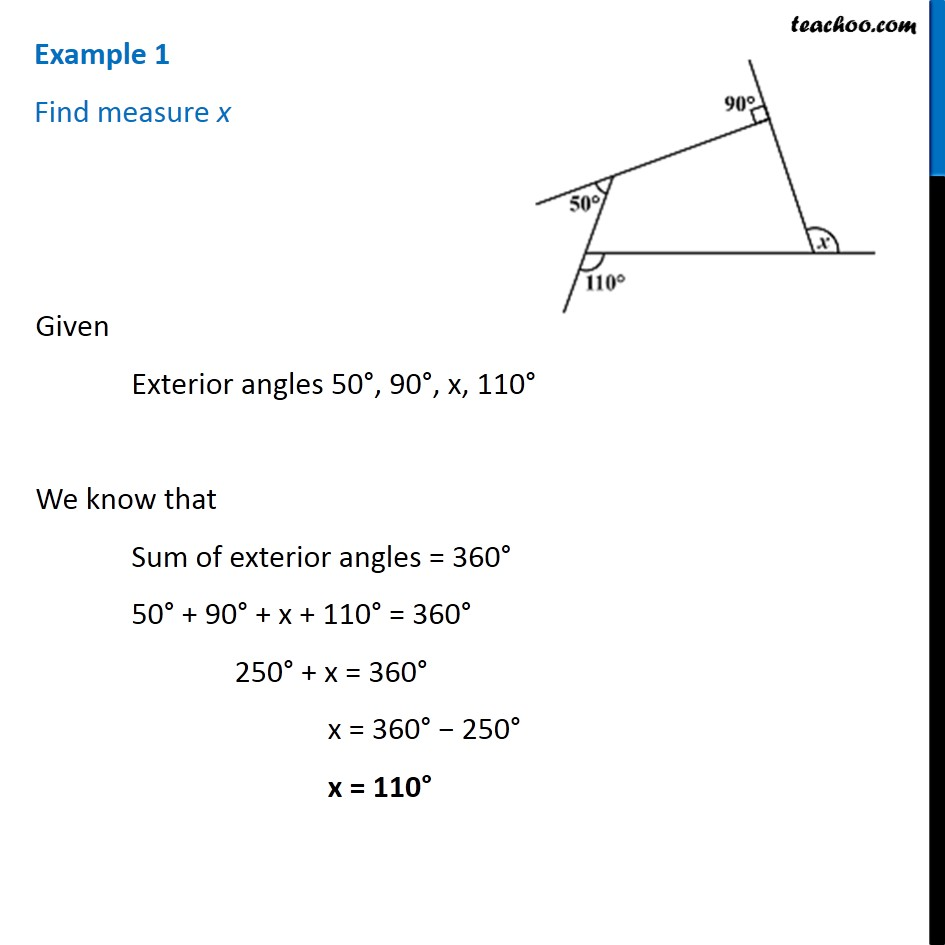Sum of Exterior Angles of Polygons### Transcript

Example 1 Find measure x Given Exterior angles 50°, 90°, x, 110° We know that Sum of exterior angles = 360° 50° + 90° + x + 110° = 360° 250° + x = 360° x = 360° − 250° x = 110°